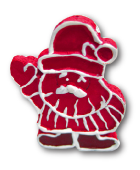### Challenge from 13. December

T-shirts

The weekend challenges can be solved without wasting points until Monday 11:59:59pm (CET). For more information see the rules of the game.

Enjoy the puzzle!

Author: Hajo Broersma

Project: 4TU.AMI

Challenge:

Sixteen pixies are standing in a circle. All of the pixies have one integer printed on their T-shirt. The number printed on every pixie's T-shirt is always (strictly) larger than the sum of the numbers on the T-shirts of the two pixies that are standing to the left of him.

What is the largest possible number of pixies with a positive number on their T-shirts?

Artwork: Frauke JansenPossible answers:

1. The largest possible number of positive numbers is 3.

2. The largest possible number of positive numbers is 4.

3. The largest possible number of positive numbers is 5.

4. The largest possible number of positive numbers is 6.

5. The largest possible number of positive numbers is 7.

6. The largest possible number of positive numbers is 8.

7. The largest possible number of positive numbers is 9.

8. The largest possible number of positive numbers is 10.

9. The largest possible number of positive numbers is 11.

10. The largest possible number of positive numbers is 12.

You have to log in to be able to submit your answer.